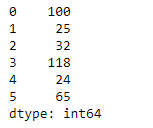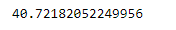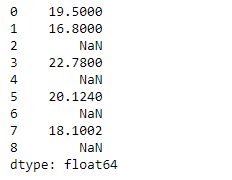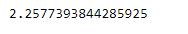# Python | Pandas Series.std()

• Last Updated : 05 Feb, 2019

Pandas series is a One-dimensional ndarray with axis labels. The labels need not be unique but must be a hashable type. The object supports both integer- and label-based indexing and provides a host of methods for performing operations involving the index.

Pandas` Series.std()` function return sample standard deviation over requested axis. The standard deviation is normalized by N-1 by default. This can be changed using the ddof argument.

Syntax: Series.std(axis=None, skipna=None, level=None, ddof=1, numeric_only=None, **kwargs)

Parameter :
axis : {index (0)}
skipna : Exclude NA/null values. If an entire row/column is NA, the result will be NA
level : If the axis is a MultiIndex (hierarchical), count along a particular level, collapsing into a scalar
ddof : Delta Degrees of Freedom. The divisor used in calculations is N – ddof, where N represents the number of elements.
numeric_only : boolean, default None

Returns : std : scalar or Series (if level specified)

Example #1 : Use `Series.std()` function to find the standard deviation of the given Series object.

 `# importing pandas as pd ` `import` `pandas as pd ` ` `  `# Creating the Series ` `sr ``=` `pd.Series([``100``, ``25``, ``32``, ``118``, ``24``, ``65``]) ` ` `  `# Print the series ` `print``(sr) `

Output :Now we will use `Series.std()` function to find the standard deviation of the given Series object.

 `# find standard-deviation along the ` `# 0th index ` `sr.std() `

Output :As we can see in the output, `Series.std()` function has successfully calculated the standard deviation the given Series object.

Example #2 : Use `Series.std()` function to find the standard deviation of the given Series object. We have some missing values in our series object, so skip those missing values.

 `# importing pandas as pd ` `import` `pandas as pd ` ` `  `# Creating the Series ` `sr ``=` `pd.Series([``19.5``, ``16.8``, ``None``, ``22.78``, ``None``, ``20.124``, ``None``, ``18.1002``, ``None``]) ` ` `  `# Print the series ` `print``(sr) `

Output :Now we will use `Series.std()` function to find the standard deviation of the given Series object.

 `# find standard-deviation along the ` `# 0th index ` `sr.std(skipna ``=` `True``) `

Output :As we can see in the output, `Series.std()` function has successfully calculated the standard deviation the given Series object. If we do not skip the missing values then the output will be `NaN`.

My Personal Notes arrow_drop_up
Recommended Articles
Page :